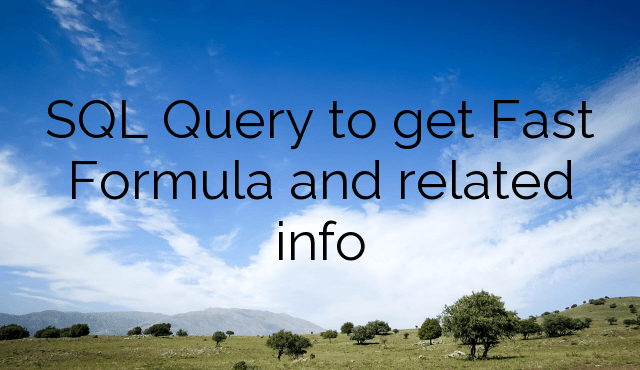SQL Query to get Fast Formula and related info

1,732 total views

Below are the SQL’s to extract Fast Formula related data from underlying tables:

Fast Formula Functions and their underlying Packages:

`SELECT f.BASE_FUNCTION_NAME ,f.description ,f.DEFINITION || '(' || listagg(fp.name || ' ' ||  decode(fp.class, 'I', 'IN', 'O', 'OUT') || ' ' ||  decode(fp.data_type, 'N', 'NUMBER', 'T', 'VARCHAR2', 'D', 'DATE'), ',')  WITHIN GROUP (ORDER BY fp.sequence_number) || ') RETURN ' || decode(f.data_type,'N','NUMBER','T','VARCHAR2','D','DATE') AS functionCallFROM ff_function_parameters fp ,FF_FUNCTIONS_VL fWHERE f.function_id = fp.function_id AND upper(f.description) NOT LIKE '%DEPRECATED%'GROUP BY f.BASE_FUNCTION_NAME ,f.description ,f.DEFINITION ,f.data_type`

Fast Formula‘s List with FF Code:

```select TO_CHAR("FF_FORMULAS_VL"."EFFECTIVE_START_DATE",'DD-MON-YYYY') as "EFFECTIVE_START_DATE",
TO_CHAR("FF_FORMULAS_VL"."EFFECTIVE_END_DATE",'DD-MON-YYYY') as "EFFECTIVE_END_DATE",
"FF_FORMULAS_VL"."BASE_FORMULA_NAME" as "BASE_FORMULA_NAME",
"FF_FORMULAS_VL"."FORMULA_NAME" as "FORMULA_NAME",
"FF_FORMULAS_VL"."DESCRIPTION" as "DESCRIPTION",
"FF_FORMULAS_VL"."EDIT_STATUS" as "EDIT_STATUS",
"FF_FORMULAS_VL"."FORMULA_TEXT" as "FORMULA_TEXT",
"FF_FORMULAS_VL"."COMPILE_FLAG" as "COMPILE_FLAG",
"FF_FORMULAS_VL"."LEGISLATION_CODE" as "LEGISLATION_CODE",
TO_CHAR("FF_FORMULAS_VL"."LAST_UPDATE_DATE",'DD-MON-YYYY') as "LAST_UPDATE_DATE",
"FF_FORMULAS_VL"."LAST_UPDATED_BY" as "LAST_UPDATED_BY",
"FF_FORMULAS_VL"."CREATED_BY" as "CREATED_BY",
TO_CHAR("FF_FORMULAS_VL"."CREATION_DATE",'DD-MON-YYYY') as "CREATION_DATE",
"FF_FORMULA_TYPES_TL"."FORMULA_TYPE_NAME" as "FORMULA_TYPE_NAME"
from "FUSION"."FF_FORMULA_TYPES_TL" "FF_FORMULA_TYPES_TL",
"FUSION"."FF_FORMULAS_VL" "FF_FORMULAS_VL"
where "FF_FORMULAS_VL"."FORMULA_TYPE_ID"="FF_FORMULA_TYPES_TL"."FORMULA_TYPE_ID"```

Extracting DBIs from a POD:

`SELECT fat.module_name ,fdg.base_group_name ,fdg.group_name ,fdi.base_user_name ,fdi.user_name ,fdi.description ,fdi.data_type ,fdi.definition_text ,fue.base_user_entity_name ,fue.description fue_des ,fr.base_route_name ,fr.multi_row_flag ,(  SELECT substr(sys.stragg(',' || base_context_name), 2) context  FROM fusion.ff_route_context_usages i   ,fusion.ff_contexts_vl j  WHERE i.context_id = j.context_id   AND i.route_id = fr.route_id  ) contexts_used ,(  SELECT substr(sys.stragg(',' || parameter_name), 2) context  FROM fusion.ff_route_parameters  WHERE route_id = fr.route_id  ) parameters ,(  SELECT substr(sys.stragg(',' || base_context_name), 2) context  FROM fusion.ff_dbi_groups_vl a   ,fusion.ff_dbi_usages b   ,fusion.ff_database_items_vl c   ,fusion.ff_contexts_vl d  WHERE a.context_id = d.context_id   AND a.dbi_group_id = b.dbi_group_id   AND b.dbi_id = c.database_item_id   AND c.user_entity_id = fue.user_entity_id  ) contexts_setFROM fusion.ff_database_items_vl fdi ,fusion.ff_dbi_usages fdu ,fusion.ff_dbi_groups_vl fdg ,fusion.fnd_appl_taxonomy_vl fat ,fusion.ff_user_entities_vl fue ,fusion.ff_routes_vl frWHERE fdi.module_id IS NOT NULL AND fdi.database_item_id = fdu.dbi_id(+) AND fdu.dbi_group_id = fdg.dbi_group_id(+) AND fdi.module_id = fat.module_id AND fdi.user_entity_id = fue.user_entity_id AND fue.route_id = fr.route_id AND fdi.module_id IS NOT NULLORDER BY module_name ,fdi.base_user_name`

Fast Formula Contexts by Formula Type:

`SELECT t.base_formula_type_name ,ttl.formula_type_name ,ttl.description ,c.base_context_nameFROM ff_formula_types_b t ,ff_formula_types_tl ttl ,ff_ftype_context_usages u ,ff_contexts_b cWHERE t.formula_type_id = u.formula_type_id AND ttl.formula_type_id = t.formula_type_id AND ttl.LANGUAGE = userenv('LANG') /*and    ttl.formula_type_name like 'Oracle%Payroll%'*/ AND c.context_id = u.context_id`

If you have any questions, please feel free to reach out to me by posting in comments section.

If you are interested in learning Fusion Technical tools go through this post

If you liked the article, please share it with your friends/ colleagues/ teammates or anyone who might also benefit from it.

The following two tabs change content below.#### Sricharan Monigari

Sricharan is a Fusion HCM Solution Architect with over 13+ years of overall experience and 5+ years of Fusion experience. He is passionate about technical aspects of Fusion HCM and writes interesting articles on HCM Extracts, HCM Data Loader, Fast Formula, BI Publisher, Integrations and Automation etc.